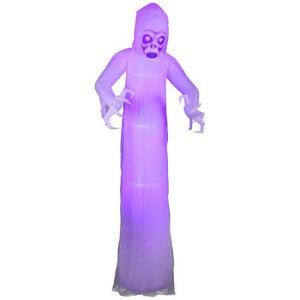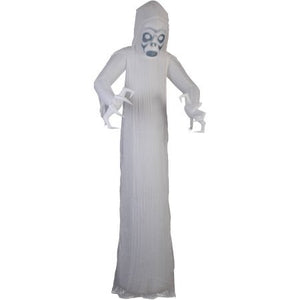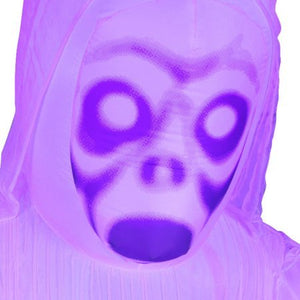# Gemmy Airblown Inflatable 12' X 4' Giant Black Light Short Circuit Ghost Halloween Decoration

Vendor
Gemmy
Regular price
Sold out
Sale price
\$200.27
Quantity must be 1 or more

• S

• e

• l

• f

• -

• i

• n

• f

• l

• a

• t

• e

• s

• i

• n

• s

• e

• c

• o

• n

• d

• s

• a

• n

• d

• c

• o

• m

• e

• s

• w

• i

• t

• h

• e

• v

• e

• r

• y

• t

• h

• i

• n

• g

• n

• e

• e

• d

• e

• d

• f

• o

• r

• e

• a

• s

• y

• o

• u

• t

• d

• o

• o

• r

• s

• e

• t

• u

• p

• Color : Black

This Airblown inflatable ghost is a scary greeting for your neighbors and guests. It lights up and self-inflates in seconds. Comes with everything needed for easy outdoor setup.